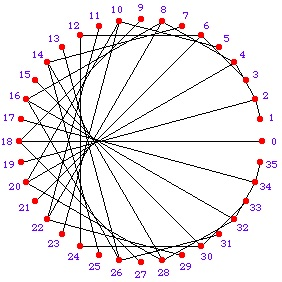Cardioid technology is used in microphones and speakers. Cardioids appear in the motion of planets and in many other ways. See this YouTube video.

To draw a cardioid, first draw a circle, then carefully mark 36 points around the circle at 10 degree intervals and number the points n = 0 to 35. You are going to draw cardioids and other envelopes by joining each point n to the point given by some function of n.

1. Join $n \to 2n$ using clock arithmetic.

You already use clock arithmetic in: 12s with months and with hours; in 60s with minutes and seconds; and also in 7’s with days of the week.

Double each number then count on round the circle and join point n to point 2n each time. For example 19 is joined to 2 because 2 times 19 is 38 and you count round the circle 2 spaces past point 36 because 38=1 x 36 + 2. Similarly 25 is joined to 14 because 2 times 25 is 50 and 50=1 x 36 + 14.

This shape is called a nephroid. Start with a base circle and a diameter of that circle. Construct the nephroid by drawing circles with centres on the base circle and tangent to the diameter of the base circle.

The cardioid and nephroid are examples of envelopes which are curves that are tangent to a family of curves at each point of contact.

If you join the positions of pairs of planets in our Solar System at different times you get these patterns. This happens because the motion of the planets around the sun is periodic. The video (link above) shows the pattern for the Earth and Venus.